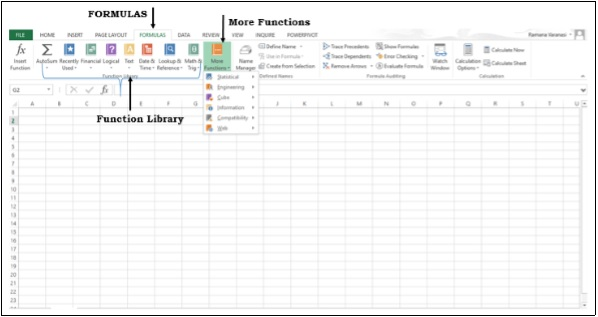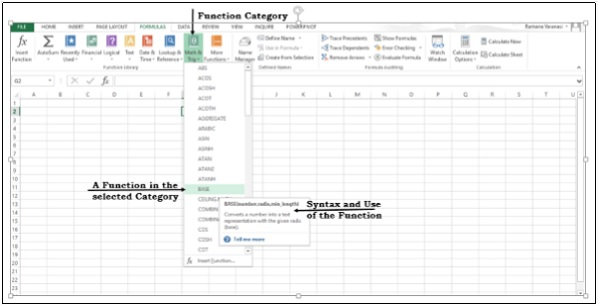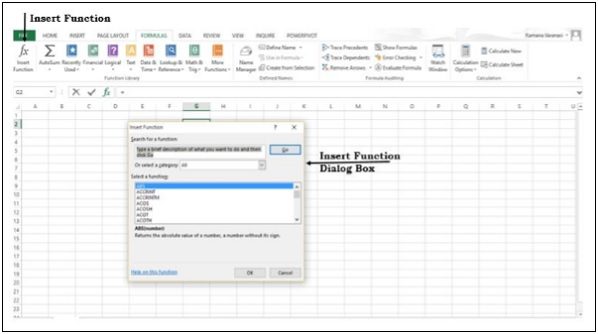# Advanced Excel - New Functions

Several new functions are added in the math and trigonometry, statistical, engineering, date and time, lookup and reference, logical, and text function categories. Also, Web category is introduced with few Web service functions.

## Functions by Category

Excel functions are categorized by their functionality. If you know the category of the function that you are looking for, you can click that category.

Step 1 − Click on the FORMULAS tab. The Function Library group appears. The group contains the function categories.

Step 2 − Click on More Functions. Some more function categories will be displayed.Step 3 − Click on a function category. All the functions in that category will be displayed. As you scroll on the functions, the syntax of the function and the use of the function will be displayed as shown in the image given below.## New Functions in Excel 2013

### Date and Time Functions

• DAYS − Returns the number of days between two dates.

• ISOWEEKNUM − Returns the number of the ISO week number of the year for a given date.

### Engineering Functions

• BITAND − Returns a 'Bitwise And' of two numbers.

• BITLSHIFT − Returns a value number shifted left by shift_amount bits.

• BITOR − Returns a bitwise OR of 2 numbers.

• BITRSHIFT − Returns a value number shifted right by shift_amount bits.

• BITXOR − Returns a bitwise 'Exclusive Or' of two numbers.

• IMCOSH − Returns the hyperbolic cosine of a complex number.

• IMCOT − Returns the cotangent of a complex number.

• IMCSC − Returns the cosecant of a complex number.

• IMCSCH − Returns the hyperbolic cosecant of a complex number.

• IMSEC − Returns the secant of a complex number.

• IMSECH − Returns the hyperbolic secant of a complex number.

• IMSIN − Returns the sine of a complex number.

• IMSINH − Returns the hyperbolic sine of a complex number.

• IMTAN − Returns the tangent of a complex number.

### Financial Functions

• PDURATION − Returns the number of periods required by an investment to reach a specified value.

• RRI − Returns an equivalent interest rate for the growth of an investment.

### Information Functions

• ISFORMULA − Returns TRUE if there is a reference to a cell that contains a formula.

• SHEET − Returns the sheet number of the referenced sheet.

• SHEETS − Returns the number of sheets in a reference.

### Logical Functions

• IFNA − Returns the value you specify if the expression resolves to #N/A, otherwise returns the result of the expression.

• XOR − Returns a logical exclusive OR of all arguments.

### Lookup and Reference Functions

• FORMULATEXT − Returns the formula at the given reference as text.

• GETPIVOTDATA − Returns data stored in a PivotTable report.

### Math and Trigonometry Functions

• ACOT − Returns the arccotangent of a number.

• ACOTH − Returns the hyperbolic arccotangent of a number.

• BASE − Converts a number into a text representation with the given radix (base).

• CEILING.MATH − Rounds a number up, to the nearest integer or to the nearest multiple of significance.

• COMBINA − Returns the number of combinations with repetitions for a given number of items.

• COT − Returns the cotangent of an angle.

• COTH − Returns the hyperbolic cotangent of a number.

• CSC − Returns the cosecant of an angle.

• CSCH − Returns the hyperbolic cosecant of an angle.

• DECIMAL − Converts a text representation of a number in a given base into a decimal number.

• FLOOR.MATH − Rounds a number down, to the nearest integer or to the nearest multiple of significance.

• ISO.CEILING − Returns a number that is rounded up to the nearest integer or to the nearest multiple of significance.

• MUNIT − Returns the unit matrix or the specified dimension.

• SEC − Returns the secant of an angle.

• SECH − Returns the hyperbolic secant of an angle.

### Statistical Functions

• BINOM.DIST.RANGE − Returns the probability of a trial result using a binomial distribution.

• GAMMA − Returns the Gamma function value.

• GAUSS − Returns 0.5 less than the standard normal cumulative distribution.

• PERMUTATIONA − Returns the number of permutations for a given number of objects (with repetitions) that can be selected from the total objects.

• PHI − Returns the value of the density function for a standard normal distribution.

• SKEW.P − Returns the skewness of a distribution based on a population: a characterization of the degree of asymmetry of a distribution around its mean.

### Text Functions

• DBCS − Changes half-width (single-byte) English letters or katakana within a character string to full-width (double-byte) characters.

• NUMBERVALUE − Converts text to number in a locale-independent manner.

• UNICHAR − Returns the Unicode character that is references by the given numeric value.

• UNICODE − Returns the number (code point) that corresponds to the first character of the text.

## User Defined Functions in Add-ins

The Add-ins that you install contain Functions. These add-in or automation functions will be available in the User Defined category in the Insert Function dialog box.• CALL − Calls a procedure in a dynamic link library or code resource.

• EUROCONVERT − Converts a number to euros, converts a number from euros to a euro member currency, or converts a number from one euro member currency to another by using the euro as an intermediary (triangulation).

• REGISTER.ID − Returns the register ID of the specified dynamic link library (DLL) or code resource that has been previously registered.

• SQL.REQUEST − Connects with an external data source and runs a query from a worksheet, then returns the result as an array without the need for macro programming.

## Web Functions

The following web functions are introduced in Excel 2013.

• ENCODEURL − Returns a URL-encoded string.

• FILTERXML − Returns specific data from the XML content by using the specified XPath.

• WEBSERVICE − Returns the data from a web service.